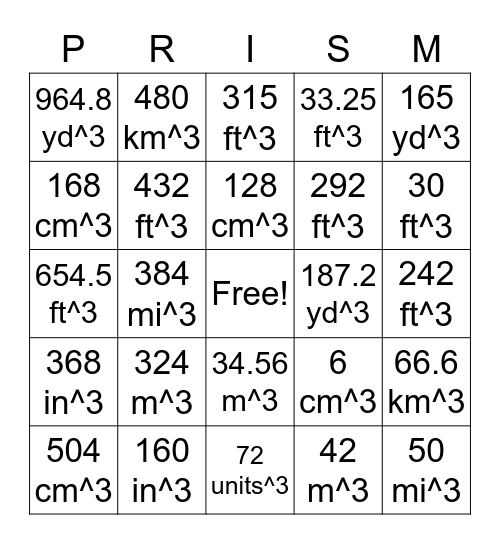# VolumesThis bingo card has a free space and 34 words: 210 mi^3, 16 in^3, 15 m^3, 36 km^3, 242 ft^3, 168 cm^3, 432 ft^3, 128 cm^3, 292 ft^3, 50 mi^3, 654.5 ft^3, 480 km^3, 42 m^3, 348 mi^3, 368 in^3, 384 mi^3, 72 units^3, 187.2 yd^3, 66.6 km^3, 504 cm^3, 160 in^3, 34.56 m^3, 33.25 ft^3, 30 ft^3, 84 km^3, 324 m^3, 315 ft^3, 216 m^3, 165 yd^3, 964.8 yd^3, 120 m^3, 6 cm^3, 335.4 m^4 and 12 mi^3.

## Play Online